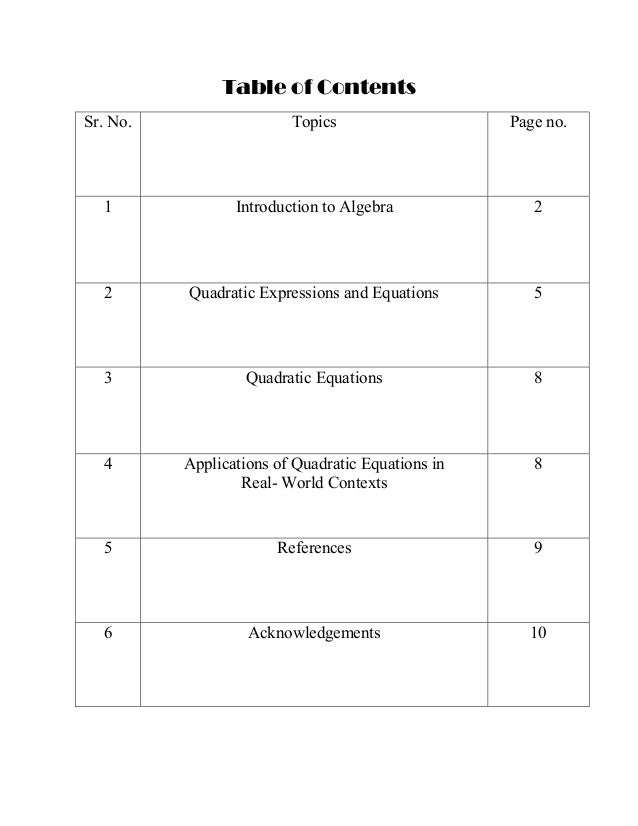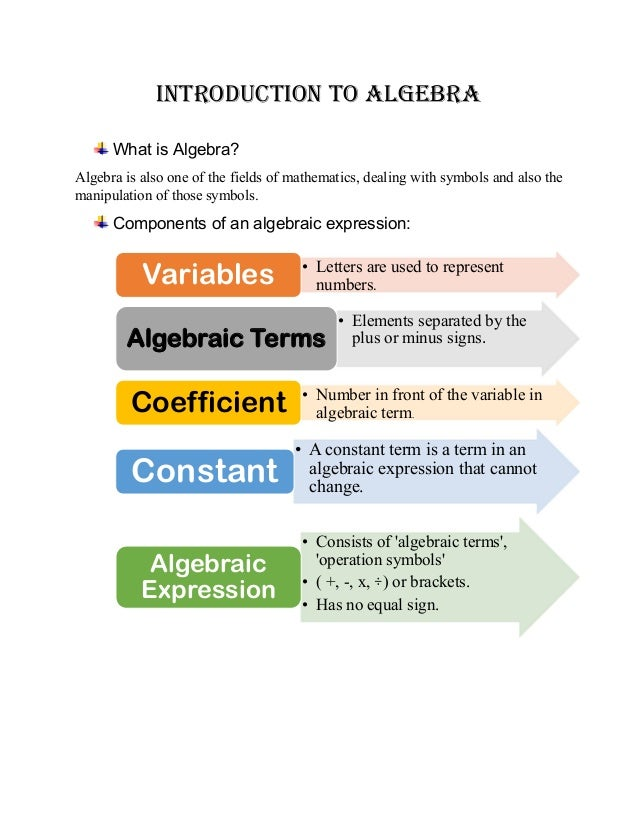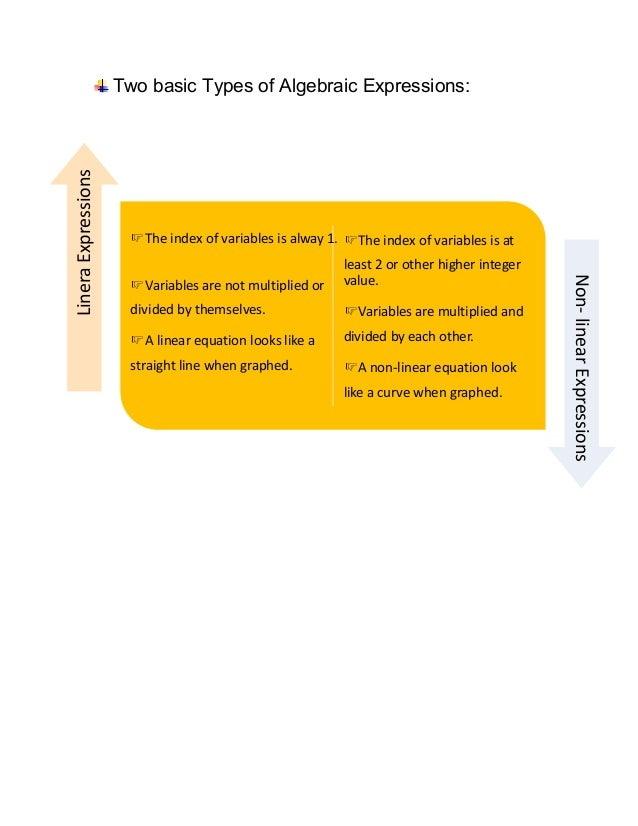Successfully reported this slideshow.Upcoming SlideShare
×

ofUpcoming SlideShare
Next

2

Share

# Algebra booklet

This booklet is a brief introduction to Algebra, one of the most main field of mathematics. Further it introduces learners to the worlds of quadratics and concepts of factorization and expansion.

See all

See all

### Algebra booklet

1. 1. Table of Contents Sr. No. Topics Page no. 1 Introduction to Algebra 2 2 Quadratic Expressions and Equations 5 3 Quadratic Equations 8 4 Applications of Quadratic Equations in Real- World Contexts 8 5 References 9 6 Acknowledgements 10
2. 2. IntroductIon to AlgebrA What is Algebra? Algebra is also one of the fields of mathematics, dealing with symbols and also the manipulation of those symbols. Components of an algebraic expression: • Letters are used to represent numbers.Variables • Elements separated by the plus or minus signs.Algebraic Terms • Number in front of the variable in algebraic term. Coefficient • A constant term is a term in an algebraic expression that cannot change. Constant • Consists of 'algebraic terms', 'operation symbols' • ( +, -, x, ÷) or brackets. • Has no equal sign. Algebraic Expression
3. 3. Two basic Types of Algebraic Expressions: The index of variables is alway 1. Variables are not multiplied or divided by themselves. A linear equation looks like a straight line when graphed. The index of variables is at least 2 or other higher integer value. Variables are multiplied and divided by each other. A non-linear equation look like a curve when graphed. LineraExpressions Non-linearExpressions
4. 4. QuAdrAtIc expressIons And eQuAtIons What are “quadratics”?  An equation where the highest exponent of the variable is power to 2.  The general form of a quadratic expression in one variable is ax2 + bx + c, where x is the variable, a, b and c are the constants and a ≠ 0. Expansion and Simplification of Quadratics  Expansion means to remove brackets from an expression. This is done by multiplying everything inside a bracket with the number outside the bracket.  This means that after solving the expression, there should be no brackets in the answer. 1) Method 1: Expansion of quadratics of the forms a(b+c), ab, and (ab)c can also be done using the 3 laws of Algebra: Distributive, Commutative and Associative laws respectively (for multiplication)
5. 5. 2) Method 2: Expansion of Quadratic Expressions can also be done using special algebraic identities. (a+b)2 and (a-b)2 are known as perfect squares while (a+b)(a-b) is called the difference of 2 squares. Factorisation of Quadratics: Factorisation is the process of expressing an algebraic expression as a product of two or more algebraic expressions. Factorisation is the reverse of expansion. 1) Method 1: We can use the multiplication frame for factorising a quadratic expression. E.g.: c2 – 9c + 20
6. 6. 2) Method 2: Like Expansion, algebraic identities can also be used for factorization of quadratics. We factorise an expression using these algebraic identities if the expression can be expressed in one of the forms on the left- hand side of the equations. 3) Method 3: Factorising the quadratic expression through middle term breaking:  6x 2 + 19x + 10 Find the product of 1st and last term (ac): 6 x 10 = 60 4) Method 4: Factorisation by grouping:  ax + bx + kay + kby = x(a + b) + ky(a + b) = (a + b)(x + ky)  (a + b)2 ≠ a2 + b2  (a – b)2 ≠ a2 – b2
7. 7. QuAdrAtIc eQuAtIons ApplIcAtIons of QuAdrAtIc eQuAtIons In reAl- World contexts  For example, in physics, quadratic models are used to solve problems involving the motion of objects such as a ball or a rocket that are projected directly upwards.  In finance, the formulation of quadratic equations to solve problems involving maximization of profit and minimization of cost helps businessmen make informed decisions.  Quadratic equations are actually used in everyday life, as when calculating areas, determining a product's profit or formulating the speed of an object.  For a parabolic mirror, a reflecting telescope or a satellite dish, the shape is defined by a quadratic equation.  Quadratic equations are also needed when studying lenses and curved mirrors.  And many questions involving time, distance and speed need quadratic equations. If two factors P and Q are such that P x Q = 0, then either P = 0 or Q = 0 or both P and Q are equal to 0. The principal can be used to solve the quadratic equations of the form ax2 + bx + c = 0, where a, b and c are constants and a ≠ 0. Many real- life problems can be modelled by quadratic functions and related problems can then be solved using quadratic equations.
8. 8. references  Oxford New Syllabus Mathematics 1, 7th Edition, Chapter 4 about Algebraic Manipulation  Oxford New Syllabus Mathematics 2, 7th Edition, Chapters 3, 4 and 5 about Quadratic and Algebraic Expressions and Equations  https://en.wikiversity.org/wiki/Basic_Laws_of_Algebra  https://www.mathsisfun.com/algebra/quadratic-equation-real- world.html  https://funwithquadratic.wordpress.com/
•#### UsmanAbubakar27

Jun. 23, 2021
•#### RameenKamran1

Nov. 22, 2020

Total views

53

On Slideshare

0

From embeds

0

Number of embeds

0

0

Shares

0